• 信号处理趣学D1——相关函数的意义&利用自相关函数消除噪声
千次阅读 多人点赞
2020-03-26 23:07:08

小虎在这里介绍了相关函数的意义和工程应用，工程应用以提取受噪声干扰的周期信号为例，并用MATLAB进行仿真。

## 什么是相关函数

相关函数(correlation function)是用来衡量两个信号的相关程度。相关函数又分为自相关函数、互相关函数和协方差函数。这里仅介绍在测试技术中较常见的两种（前两种）。

### 自相关函数

自相关函数(autocorrelation function)是用来衡量统一信号在不同时刻取值的相关程度。通过这种分析，可以分析出信号中的噪声并加以去除；或者从畸变的波形中分离出基波和谐波等。可以将时移前后的信号当成两个信号分析。这是仿真理解demo
R x ( τ ) = lim ⁡ T → ∞ 1 T ∫ 0 T x ( t ) x ( t + τ ) d t R_x(\tau)=\lim \limits_{T \to \infty } \frac{1}{T} \int_0^Tx(t)x(t+\tau)dt

### 互相关函数

互相关函数(crosscorrelation function)用来衡量两个信号之间的相关程度和取值依赖程度。对于一个理想的测试系统的输入输出信号求相关函数，测试结果完美的情况下，互相关函数取最大值时的 τ \tau 时为等于系统的滞后时间，因为这说明信号并没有损失或收到噪声干扰，给输入信号加上的延时 t a u tau 后与输出的信号一模一样，所以相关程度为完全相同。这是仿真理解demo
R x y ( τ ) = lim ⁡ T → ∞ 1 T ∫ 0 T x ( t ) y ( t + τ ) d t R_{xy}(\tau)=\lim \limits_{T \to \infty } \frac{1}{T} \int_0^Tx(t)y(t+\tau)dt
另有性质之一 R x y ( − τ ) = R y x ( τ ) R_{xy}(-\tau)=R_{yx}(\tau) ，所以当 y ( t ) y(t) 比较复杂时可以采用这种方法转换为对 x ( t ) x(t) 时延来计算。

## 相关函数提取周期信号原理

对机器进行噪声诊断时，噪声通常由随机、大量且大小近似相等影响因素叠加而成。随机噪声的自相关函数将出现规则的周期信号，幅值一般比正常噪声的幅值要大。当将变速箱各个机轴的转速化成频率与自相关函数波动频率比较，可以确定机械轴的好坏。或者利用自相关函数分析去除噪声得到周期函数的周期。

## 具体例子——MATLAB仿真示例

提取受到噪声干扰的周期函数 x = s i n ( w 0 t ) x=sin(w_0t) 的自相关函数,分析原周期函数的周期。

### 物理意义

结果如下图。为了便于计算，将 w 0 = 2 π f 0 w_0=2\pi f_0 计算。• 可以看到，每过一个周期，原周期函数重合，相关程度最高；每过半个周期，原周期函数互为相反数，相关程度为负的最大，这里我设的函数周期正好是0.2s（即200ms），可以很好的从自相关函数-滞后时间图像中看出。
• 横坐标0的时候，时移为零，函数图像重合，时移前后的两个函数完全相同，相关程度最大，所以出现了0时刻的正的尖峰。
• 另外，噪声的干扰还是可以在自相关函数-滞后时间的图中看出的，可以看到曲线不是完美的曲线，而是由波动的折线拟合而成的，这是随机噪声带来的影响，但是不影响我们分析周期函数的周期。

### 代码分析

1. 参数设置
取6000采样长度，1000采样频率，t为采样间隔， w 0 = 2 π f 0 = 10 π w_0=2\pi f_0=10\pi
n=6000;
fs=1000;
t=(0:n-1)/fs;
f0=5;

1. 函数建立
x为周期为 1 / f 0 1/f_0 的正弦周期函数，z为x受到随机噪声干扰后的函数。
x=sin(2*pi*f0*t);
z=x+randn(size(x));

1. 自相关函数求解
R是求得的自相关函数；tau时延的值，截取了包括0在内的1+600x2个点。600时时延的区间，以傅里叶复指数形式表示的频谱是双边谱，傅里叶三角函数表示形式是单边谱，这里为前者，其实是后者的一分为二，这完全是数学计算的结果，没有任何实际物理意义。'coeff’归一化求得是自相关函数，‘biased’是有偏估计，'unbiased’是无偏估计。
[R,tau]=xcorr(z,600,'coeff');

1. 作图
用两个画板画出受噪声干扰的函数和改函数的自相关函数。
subplot(2,1,1);
plot(t(1:1000),z(1:1000));
xlabel('时间/s');
ylabel('幅值');
subplot(2,1,2);
plot(tau,R);
xlabel('滞后');
ylabel('自相关函数');

1. 完整代码
n=6000;
fs=1000;
t=(0:n-1)/fs;
f0=5;
x=sin(2*pi*f0*t);
z=x+randn(size(x));
[R,tau]=xcorr(z,600,'coeff');
subplot(2,1,1);
plot(t(1:1000),z(1:1000));
xlabel('时间/s');
ylabel('幅值');
subplot(2,1,2);
plot(tau,R);
xlabel('滞后');
ylabel('自相关函数');


## 参考文献

张春华等，工程测试技术基础第二版

## 更多

信号处理趣学D0——系列专栏的说明与目录测试工程师 matlab 傅立叶分析
更多相关内容
• 互相关应用的方向等，互相关应用的方向等，互相关应用的方向等
• ## 互相关函数的实际应用

万次阅读 多人点赞 2018-04-10 20:46:50
互相关函数定义令f1(t), f2(t) 为能量信号，一般情况可以是时间的复函数，称：为f1(t)和f2(t) 的互相关函数应用：①噪声背景下提取有用信息上图为信号的传递系统，其中n（t）为噪声。但只有系统对输入的响应是...

### 互相关函数定义

令f1(t), f2(t) 为能量信号，一般情况可以是时间的复函数，称：为f1(t)和f2(t) 的互相关函数。

应用：

①在噪声背景下提取有用信息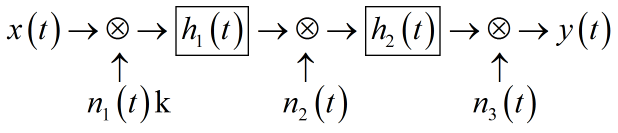上图为信号的传递系统，其中n（t）为噪声。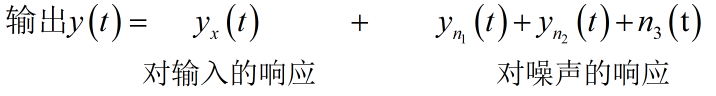但只有系统对输入的响应是有用的。

通过一个互相关器，输出就相当于 x(t) 与 Yx(t) , Yn1(t) , Yn2(t) , n3(t)分别求互相关函数再叠加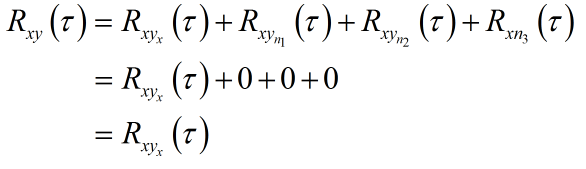总结为一句话就是“同频相关，不同频不相关”。

做到这一步时已经由互相关器得出了Rxyx（τ）分离出了噪声，下一步就是从分离信号中提取有用信息。

要分离有用信息先要知道两信号互相关函数的具体形式，下面以两正弦信号为例。

“已知两个信号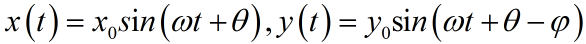，这两个信号的互相关函数是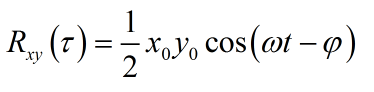”（求解过程省略）

再回到提取有用信号这个目标中，已知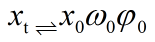由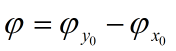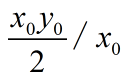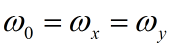解得 y(t) 即有用信息。

②求匀速钢板的运动速度

互相关函数也可以用来求轧钢厂生产线上钢板的运动速度，如图所示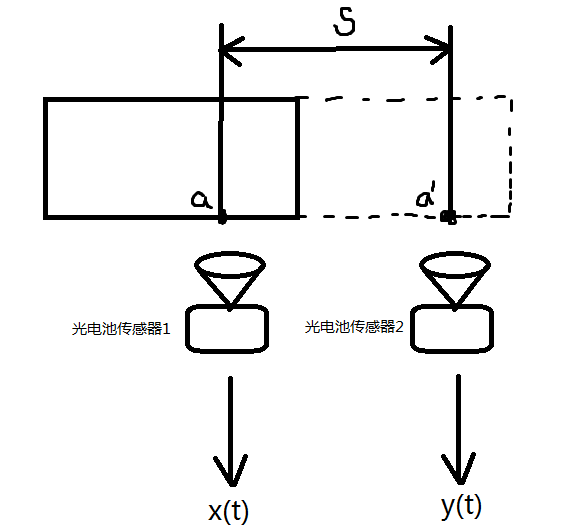钢板a点贴有反光片，当a点经过传感器1时，放大镜将光点信息传送到光电池传感器并输出x(t)，同理，a点经过传感器2时，产生一个y(t)，因为两传感器检测的时同一点的信号，故y(t)是x(t)的一个时移，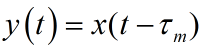，且钢板运动速度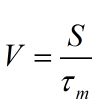互相关函数式为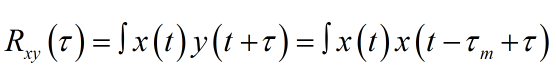通过上式可知τ=τm时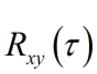取最大值，所以分析图像即知峰值点对应的τ就是τm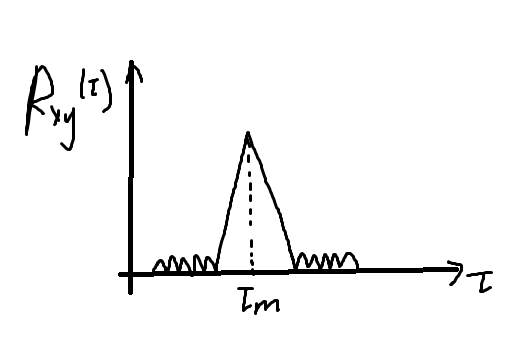求出 τm 便可通过求出 V 。

③求输油管裂损的位置

原理与②类似，都是通过τm来解决问题。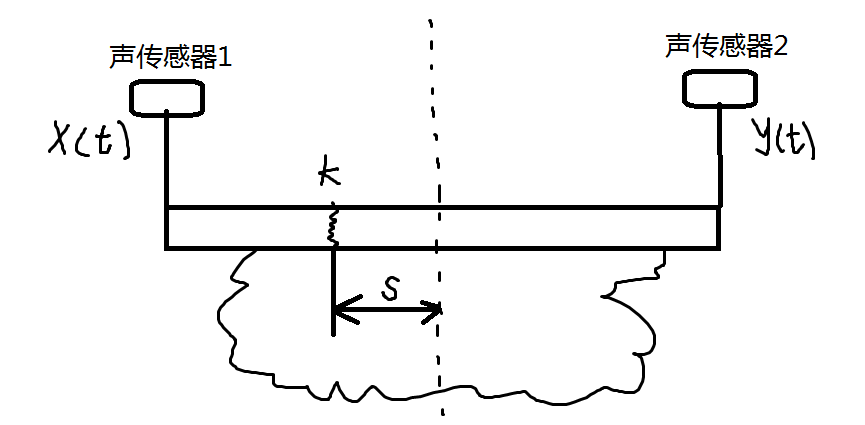当漏油面积较大时很难从视觉上判断漏油点，这时根据漏油面积找到两个极限测量点（漏油点一定在两测量点之间），根据漏油的滴答声到两传感器之间的时间差来判断漏油的具体位置。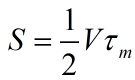，    V为常数，由管道材质决定，公式的推导过程本文省略。τm的求解方式与用途②中的τm求解方式相同，由此便可解出S。

展开全文• 作者结合自己学习实践，从以下三个方面介绍对比了自相关与互相关 1. 定义 2. 从其他角度对于相关的理解 3. 在工程实践应用
• 利用MATLAB程序互动理解互相关函数，随意按键移动信号2，造成不同时延得到不同的相关程度。

## 背景介绍

利用MATLAB程序互动理解互相关函数，相关函数的工程应用可以看这里
随意按键移动信号2，造成不同时延得到不同的相关程度。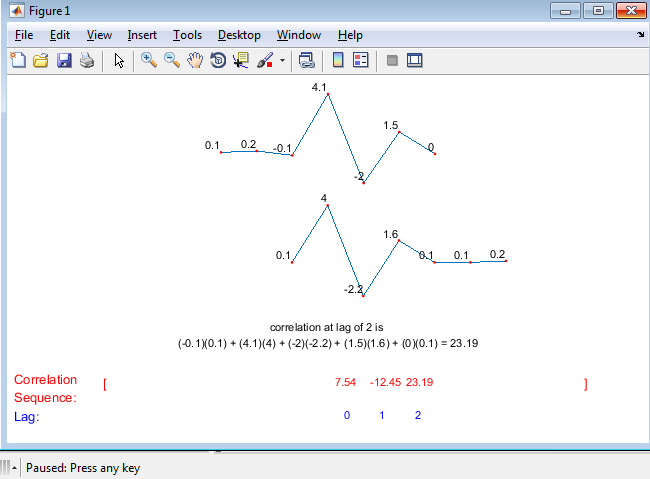## 完整代码

% A demonstration of cross correlation in action.
% Hit the space bar during the demo to execute
%
clc;close all
a = [0.1 0.2  -0.1 4.1 -2 1.5 0 ];
b = [0.1 4 -2.2 1.6 0.1 0.1 0.2];

len = length(a);
if(len ~= length(b))
error('vectors supplied must be the same length');
end
figure
set(gcf, 'position', [ 285   347   642   367]);

max_amp = max([max(a) max(b)]);
min_amp = min([min(a) min(b)]);

plot_h = 0.25;
text_h = 0.1;
ax1 = subplot(2,1,1);
pl1_line = plot(a);
labels1 = text([1:len], a , num2str(a'), 'VerticalAlignment','bottom', ...
'HorizontalAlignment','right','fontsize',8);
hold on; pl1_dot = plot(a,'r.');
xlim([1 len])
ylim([min_amp max_amp])

set(ax1,'position', [(1/3) 0.95-plot_h (1/3) plot_h])
set(ax1,'visible','off')

ax2 = subplot(2,1,2);
pl2_line = plot(b);
labels2 = text([1:len], b , num2str(b'), 'VerticalAlignment','bottom', ...
'HorizontalAlignment','right','fontsize',8);
hold on; pl2_dot = plot(b,'r.');
xlim([1 len])
ylim([min_amp max_amp])
set(ax2,'visible','off')
set(ax2,'position', [(1/3) 0.9-plot_h*2 (1/3) plot_h])

str = '';
for k = 1: len
str = [str '(' num2str(a(k)) ')(' num2str(b(k)) ') + '];
end
str(end-1)  = '=';
str = [str num2str(sum(a.*b))];
r_ba = xcorr(a,b);

corr_calc_text = annotation('textbox',  [0 0.85-plot_h*2-text_h 1 text_h], 'linestyle','none','horizontalalignment','center' ,'string', {'correlation at zero lag is ' str}, 'fontsize', 8);

annotation('textbox',  [0.5 0.8-plot_h*2-text_h*2 1 text_h], 'linestyle','none','horizontalalignment','left' ,'string', sprintf('%.2f',r_ba(len)),'color','red', 'fontsize', 8);

pause

x_inc= (1/3)/(len-1);
for k = 1:len-1

str = '';
for m = 1: len-k
str = [str '(' num2str(a(m+k)) ')(' num2str(b(m)) ') + '];
end
str(end-1)  = '=';
str = [str num2str(r_ba(len+k))];

set(corr_calc_text,'string', {['correlation at lag of ' num2str(k) ' is '] str}, 'fontsize', 8);

set(ax2,'position', [(1/3)+k*x_inc 0.9-plot_h*2 (1/3) plot_h])

annotation('textbox',  [0.5+x_inc*k 0.8-plot_h*2-text_h*2 1 text_h], 'linestyle','none','horizontalalignment','left' ,'string', sprintf('%.2f',r_ba(len+k)),'color','red', 'fontsize', 8);
if(k ==1)
pause
annotation('textbox',  [0.5 0.01 1 text_h], 'linestyle','none','horizontalalignment','left' ,'string', ['   0'] ,'color','blue', 'fontsize', 8);
annotation('textbox',  [0.001 0.01 1 text_h], 'linestyle','none','horizontalalignment','left' ,'string', ['Lag:'] ,'color','blue');
annotation('textbox',  [0.5+x_inc*(len) 0.8-plot_h*2-text_h*2 1 text_h], 'linestyle','none','horizontalalignment','left' ,'string', ']' ,'color','red');
annotation('textbox',  [0.5-x_inc*(len-1)-x_inc/2 0.8-plot_h*2-text_h*2 1 text_h], 'linestyle','none','horizontalalignment','left' ,'string', '[' ,'color','red');
annotation('textbox',  [0.001 0.8-plot_h*2-text_h*2 1 text_h], 'linestyle','none','verticalalignment','middle','horizontalalignment','left' ,'string', {'Correlation' 'Sequence:'} ,'color','red');

end
annotation('textbox',  [0.5+x_inc*k 0.01 1 text_h], 'linestyle','none','horizontalalignment','left' ,'string', ['   ' num2str(k)] ,'color','blue', 'fontsize', 8);

pause
end

for k = 1:len-1

str = '';
for m = 1: len-k
str = [str '(' num2str(a(m)) ')(' num2str(b(m+k)) ') + '];
end
str(end-1)  = '=';
str = [str num2str(r_ba(len-k))];

set(corr_calc_text,'string', {['correlation at lag of ' num2str(-1*k) ' is '] str}, 'fontsize', 8);

set(ax2,'position', [(1/3)-k*x_inc 0.9-plot_h*2 (1/3) plot_h])
annotation('textbox',  [0.5-x_inc*k 0.8-plot_h*2-text_h*2 1 text_h], 'linestyle','none','horizontalalignment','left' ,'string', sprintf('%.2f',r_ba(len-k)),'color','red', 'fontsize', 8);
annotation('textbox',  [0.5-x_inc*k 0.01 1 text_h], 'linestyle','none','horizontalalignment','left' ,'string', ['   ' num2str(k*-1)] ,'color','blue', 'fontsize', 8);

pause
end

% Uncomment the next two lines if you would like to see a plot of the
% correlation sequence
% [corr_seq lags] =  xcorr(a,b);
% plot(lags,corr_seq)
% xlabel('lags');ylabel('correlation measure');

展开全文matlab
• 互相关测向法低信噪比时检测不到相关峰,无法实现有效的方位估计。...仿真结果表明重构的互相关函数较原互相关函数,其相 关峰较最高伪峰的相对值提高了约4.9 dB,从而可以更好地实现时延估计。方位估计结果显示此
• 文章目录相关函数1 互相关和自相关函数的定义2 相关与卷积的...实函数f1(t)f_1(t)f1​(t)和f2(t)f_2(t)f2​(t)，如为能量有限信号，它们之间的互相关函数定义为: (注:R12，R21R_{12}，R_{21}R12​，R21​下脚数字(1

# 相关函数

## 1 互相关和自相关函数的定义

比较某信号与另一延时 τ τ 的信号之间的相似度，需要引入相关函数的概念。相关函数是鉴别信号的有力工具，被广泛应用于雷达回波的识别，通信同步信号的识别等领域。相关函数也称为相关积分，它与卷积的运算方法类似。

实函数 f 1 ( t ) f_1(t) f 2 ( t ) f_2(t) ，如为能量有限信号，它们之间的互相关函数定义为:(注: R 12 ， R 21 R_{12}，R_{21} 下脚数字(12,21),前面数字代表的信号领先 τ τ )

互相关函数是两信号之间时间差 τ τ 的函数。一般 R 12 ( τ ) ≠ R 21 ( τ ) R_{12}(τ)≠ R_{21}(τ)如果 f 1 ( t ) f_1(t) f 2 ( t ) f_2(t) 是同一信号，可记为 f ( t ) f(t) ，这时无需区分 R 12 R_{12} R 21 R_{21} ，用 R ( τ ) R(τ) 表示，称为自相关函数。即 ：容易看出，对自相关函数有：可见，实函数 f ( t ) f(t) 的自相关函数是时移 τ τ 的偶函数。

## 2 相关与卷积的比较函数 f 1 ( t ) f_1(t) f 2 ( t ) f_2(t) 卷积的表达式为：
f 1 ( t ) ∗ f 2 ( t ) = ∫ − ∞ ∞ f 1 ( τ ) f 2 ( − ( τ − t ) ) d τ = ∫ − ∞ ∞ f 1 ( τ ) f 2 ( t − τ ) d τ f_{1}(t) * f_{2}(t)=\int_{-\infty}^{\infty} f_{1}(\tau) f_{2}(-(\tau-t)) d \tau=\int_{-\infty}^{\infty} f_{1}(\tau) f_{2}(t-\tau) d \tau

为了便于与卷积函数进行比较，我们将互相关函数定义式中的变量 t t τ τ 进行互换，可将实函数 f 1 ( t ) f_1(t) f 2 ( t ) f_2(t) 的互相关函数写为：

R 12 ( t ) = ∫ − ∞ ∞ f 1 ( τ ) f 2 ( τ − t ) d τ R_{12}(t)=\int_{-\infty}^{\infty} f_{1}(\tau) f_{2}(\tau-t) d \tau

两种运算的不同之处卷积开始时需要将 f 2 ( τ ) f_2(τ) 反折 f 2 ( − τ ) f_2(-τ) ，而相关运算则不需反折，仍为 f 2 ( τ ) f_2(τ) 。其他的移位、相乘和积分的运算方法相同根据卷积的定义可见由上式可知，若 f 1 ( t ) f_1(t) f 2 ( t ) f_2(t) 均为实偶函数，则卷积与相关的形式完全相同

《工程信号与系统》作者：郭宝龙等
国家精品课程：信号与系统 ，中国大学MOOC，郭宝龙，朱娟娟

展开全文• 《matlab电气工程中应用》由会员分享，可在线阅读，更多相关《matlab电气工程中应用(8页珍藏版)》请人人文库网上搜索。1、MATLAB电气应用前言Malab的概述MATLAB是矩阵实验室(Matrix Laboratory)的...
• ## 自相关与互相关

千次阅读 多人点赞 2020-04-28 20:27:01
自相关（Autocorrelation），也叫序列相关，是一个信号与其自身不同时间点的互相关。非正式地来说，自相关是对同一信号不同时间的两次观察，通过对比来评判两者的相似程度。自相关函数就是信号x(t)和它的时移...
• 相关（autocorrelation），也称为串行相关（serial correlation），是信号与自身的延迟副本之间的相关关系，它是延迟的函数。 非正式地，这是观察之间的相似性，是它们之间时间滞后的函数。 自相关分析是一种...
• 对于做科研工作或工程应用的人来说，拿到一列或数列随机信号观测序列，首先想到的就是看看它们的自相关、互相关函数，自功率谱、互功率谱密度函数估计吧。这一篇对上一篇各数据矩阵TW4615_12、TW2672_12、GY2672_...
• 火箭军工程大学博士生招生考试初试业务课考试大纲火箭军工程大学研究生招生办公室二〇一七年八月目 录TOC \o "1-3" \h \z \u 1001公共英语 - 1 -2001矩阵理论 - 2 -2002数理统计与随机过程 - 3 -2003计算方法 - 4 -...
• 信号相关函数的概念 信号相关分析 (Cross-correlation) 是一种分析两个信号之间或一个信号自身的时间依存关系和相似程度的方法 ρxy(τ)=∫−∞+∞x(t)y(t−τ)dt[∫−∞+∞x2(t)dt∫−∞+∞y2(t)dt]1/2\rho_{xy}...
• 提出一种基于降采样的固定时延估计算法，该算法进行时延估计之前先对语音信号进行白噪声化和降采样，有效抑制互相关函数中的局部极大值旁瓣，克服了传统归一化互相关函数时延估计算法应用于声学回波对消系统时...
• pdf扫描文件共259页书名:MATLAB振动信号处理应用出版社:中国水利水电出版社定价:32条形码:9787508426808ISBN:ISBN 7-5084-2680-0作者:王济,胡晓出版日期:2006-1-1简介:本书主要讲述借助简单、高效、功能强大的...
• 从盲源信号分离后非高斯性最大化出发,提出了一种基于经验特征函数的盲源信号分离方法。...采用新的盲 源信号分离方法对管道破坏产生的实际声发射信号进行分离,可将破坏点互相关定位精度提高到3%以上。
• 深入理解傅里叶变换的性质：实函数、卷积、相关、功率谱、频响函数 1实函数傅里叶变换的性质 1.1实函数傅里叶变换的性质 所以，实函数x(t)的傅里叶变换X(w)的共轭 X*(w)=X(-w) 1.2实偶函数傅里叶变换的性质 1.2实...傅里叶变换
• !"# 文章编号：!$$% & ’((()$$()$* &$!"# & $) 收稿日期：)$$( &$" &...文章分析了互相关函数求解信号相位差的原理，并介绍了 ,-./-0 下求解信号相位差的具体方法。 关键词：...
• TF-IDF特征选择时的误区、CHI Square和Information Gain特征选择时的应用 ） 二、 数据预处理用到的 （ 4. Dataset transformations ） ： ...
• 看了MATLAB关于xcorr的help文档没搞清楚什么意思，只够看到一篇不错的文章，通过简单事例即可了解其应用。 输入的是一个简单一维序列x=[1，2，3] 第一：缺省注释项，[a,b]=xcorr(x),通过该命令计算的结果为：a=3 8...matlab
• 从傅里叶级数到基于互相关运算的声音测距【学习笔记】傅里叶变换傅里叶级数离散傅里叶变换(DFT)快速傅里叶变换(FFT)单位根及其性质单位根如何加速DFT运算 傅里叶变换 傅里叶级数 任何周期函数都可以用正弦函数和余弦...傅立叶分析 算法 卷积 fft
• 为此 ,提出了一种高精度的非线性 剩余静校正方法 ,该方法以互相关值最大为目标函数 ,采用了逐步缩小范围的郭涛算法进行剩余静校正 量的全局寻优。通过理论模型和低信噪比资料的测试表明 ,该方法优于传统的剩余静校正...
• matlab查找相关函数时，找到了xcov函数和xcorr函数，仔细看了帮助文档，发现虽然计算公式不一样，但是两个函数用法相同，计算出的值也相同，于是翻了一圈百度后，找到了这个文档，讲的很细，转载下来以便今后...matlab
• 信号处理，窗函数是一种除给定区间之外取值均为0的实函数。譬如：给定区间内为常数而区间外为0的窗函数被形象地称为矩形窗。任何函数与窗函数之积仍为窗函数，所以相乘的结果就像透过窗口“看”其他函数...加窗分帧 矩形窗 汉明窗
• 从本质上来讲，特征工程是一个表示和展现数据的过程，实际工作，特征工程旨在去除原始数据的杂质和冗余，设计更高效的特征以刻画求解的问题与预测模型之间的关系。数据和特征决定了机器学习的上限，而模型和...机器学习 特征
• MATLAB电路与信号及控制理论应用來源:聯網2010-07-06 12:36:08評論版权信息书 名: MATLAB电路与信号及控制理论应用作者：陈晓平 毛彦欣出版社：中国科技大学出版时间： 2008ISBN: 9787312021923开本：...
• 《2015年电大本科C语言程序设计A期末考试复习资料.doc》由会员分享，可免费在线阅读全文，更多与《2015年电大本科C语言程序设计A期末考试复习资料(V5.1)》相关文档资源请帮帮文库(www.woc88.com)数亿文档库存里...
• Axure RP Pro - 相关问题 - 与IFrame部件的页面操作
• 浅谈实际工程中关于特征选择的一些心得体会关于特征选择方法的总结多级特征选择方法综合特征打分方法 关于特征选择方法的总结 原始特征数据集经过评价函数（过滤法、封装法、嵌入法）直接进行筛选； 原始特征数据...python 机器学习...